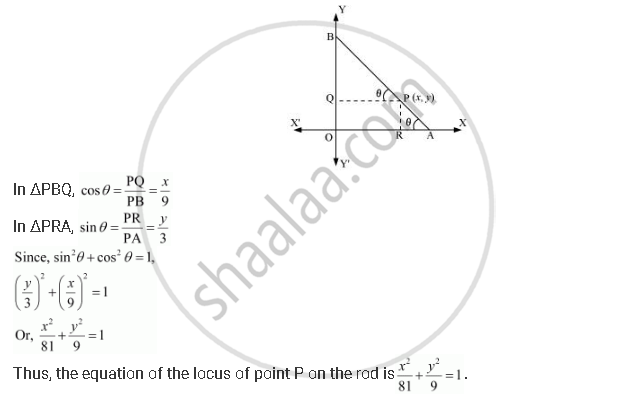CBSE (Arts) Class 11CBSE
Share

# A Rod of Length 12 Cm Moves with Its Ends Always Touching the Coordinate Axes. Determine the Equation of the Locus of a Point P on the Rod, Which is 3 Cm from the End in Contact with the X-axis. - CBSE (Arts) Class 11 - Mathematics

ConceptEllipse Relationship Between Semi-major Axis, Semi-minor Axis and the Distance of the Focus from the Centre of the Ellipse

#### Question

A rod of length 12 cm moves with its ends always touching the coordinate axes. Determine the equation of the locus of a point P on the rod, which is 3 cm from the end in contact with the x-axis.

#### Solution

Let AB be the rod making an angle θ with OX and P (x, y) be the point on it such that AP = 3 cm.

Then, PB = AB – AP = (12 – 3) cm = 9 cm [AB = 12 cm]

From P, draw PQ⊥OY and PR⊥OXIs there an error in this question or solution?

#### APPEARS IN

Solution A Rod of Length 12 Cm Moves with Its Ends Always Touching the Coordinate Axes. Determine the Equation of the Locus of a Point P on the Rod, Which is 3 Cm from the End in Contact with the X-axis. Concept: Ellipse - Relationship Between Semi-major Axis, Semi-minor Axis and the Distance of the Focus from the Centre of the Ellipse.
S